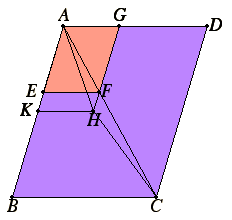# Proposition 26

If from a parallelogram there is taken away a parallelogram similar and similarly situated to the whole and having a common angle with it, then it is about the same diameter with the whole.

From the parallelogram ABCD let there be taken away the parallelogram AF similar and similarly situated to ABCD, and having the angle DAB common with it.

I say that ABCD is about the same diameter with AF.I.31

For suppose it is not, but, if possible, let AHC be the diameter. Produce GF and carry it through to H. Draw HK through H parallel to either of the straight lines AD or BC.

VI.24

Since, then, ABCD is about the same diameter with KG, therefore DA is to AB as GA is to AK.

But also, since ABCD and EG are similar, therefore DA is to AB as GA is to AE. Therefore GA is to AK as GA is to AE.

Therefore GA has the same ratio to each of the straight lines AK and AE.

V.9

Therefore AE equals AK the less equals the greater, which is impossible.

Therefore ABCD cannot fail to be about the same diameter with AF. Therefore the parallelogram ABCD is about the same diameter with the parallelogram AF.

Therefore, if from a parallelogram there is taken away a parallelogram similar and similarly situated to the whole and having a common angle with it, then it is about the same diameter with the whole.

Q.E.D.

## Guide

This proposition is the converse of VI.24. It is used in the proofs of the next three and for a few propositions in Book X.